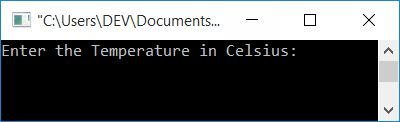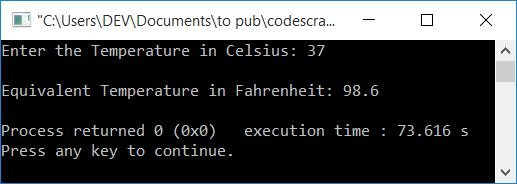# C++ program to convert Celsius to Fahrenheit

In this article, you will learn and get code on Celsius to Fahrenheit temperature conversion in C++. The program is written both with and without the use of functions.

### Celsius to Fahrenheit Conversion Formula

To convert any temperature from degrees Celsius to degrees Fahrenheit, use the following formula:

`F = (C * 1.8) + 32`

where F indicates the value of Fahrenheit and C indicates the value of Celsius.

If you're curious to know where this formula came from, you can refer to The Celsius to Fahrenheit Formula Explained.

## Centigrade to Fahrenheit in C++

To convert temperature from Celsius (or centigrade) to Fahrenheit in C++ programming, you have to ask the user to enter the temperature in Celsius. And then convert it into its equivalent value in Fahrenheit using the formula given above, as shown in the program given below:

```#include<iostream>
using namespace std;
int main()
{
float celsius, fahrenheit;
cout<<"Enter the Temperature in Celsius: ";
cin>>celsius;
fahrenheit = (celsius*1.8)+32;
cout<<"\nEquivalent Temperature in Fahrenheit: "<<fahrenheit;
cout<<endl;
return 0;
}```

This program was build and run under Code::Blocks IDE. Here is its sample run:Now supply the temperature input in degrees Celsius, say 37, and press the ENTER key to print its equivalent value in degrees Fahrenheit, as shown here in the following output:### Celsius to Fahrenheit in C++, using a user-defined function

The CelsiusToFahrehneit() user-defined function in this program converts any temperature from Celsius to Fahrenheit. This function takes a Celsius value as its argument and returns its equivalent value in Fahrenheit.

```#include<iostream>
using namespace std;
float CelsiusToFahrehneit(float);
int main()
{
float celsius, fahrenheit;
cout<<"Enter the Temperature in Celsius: ";
cin>>celsius;
fahrenheit = CelsiusToFahrehneit(celsius);
cout<<endl<<celsius<<"\370C = "<<fahrenheit<<"\370F";
cout<<endl;
return 0;
}
float CelsiusToFahrehneit(float celsius)
{
return ((celsius*1.8)+32);
}```

Here is an example of a run with user input 37:Note: 370 is the octal index of the ASCII code for the degree symbol (o). Therefore, \370 is used to print the degree symbol on output.

Note: C and F are the symbols used for Celsius and Fahrenheit, respectively.

#### The same program in different languages

C++ Quiz

« Previous Program Next Program »